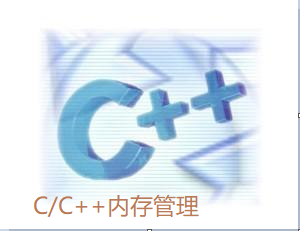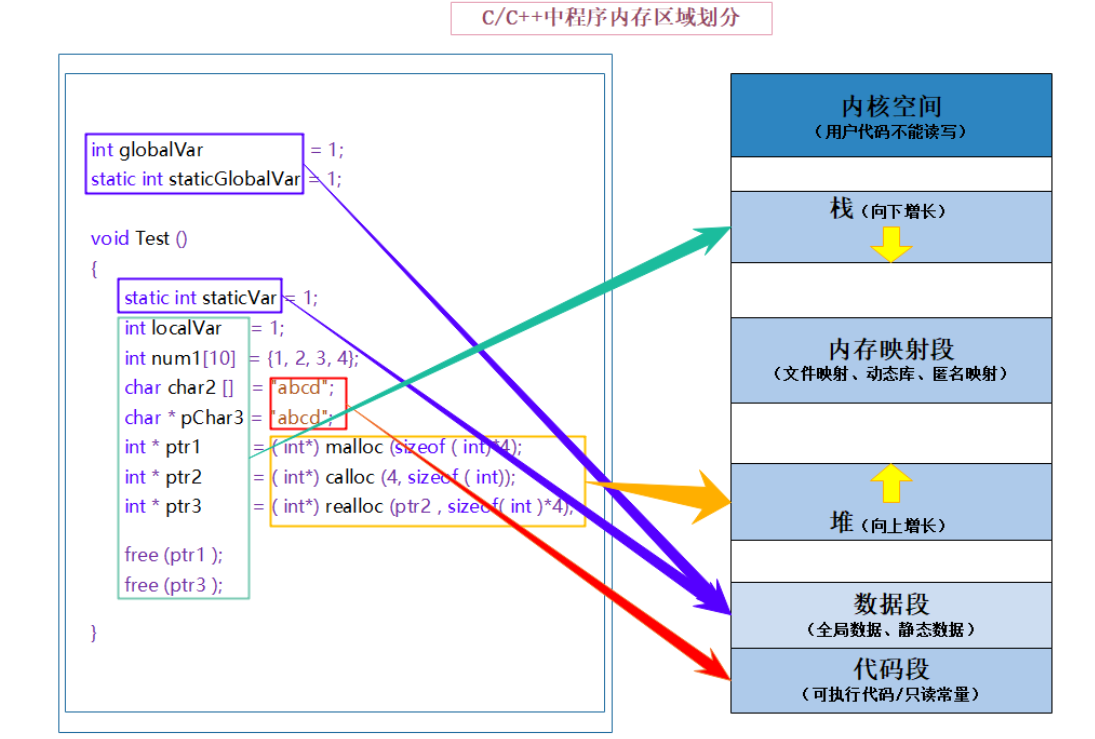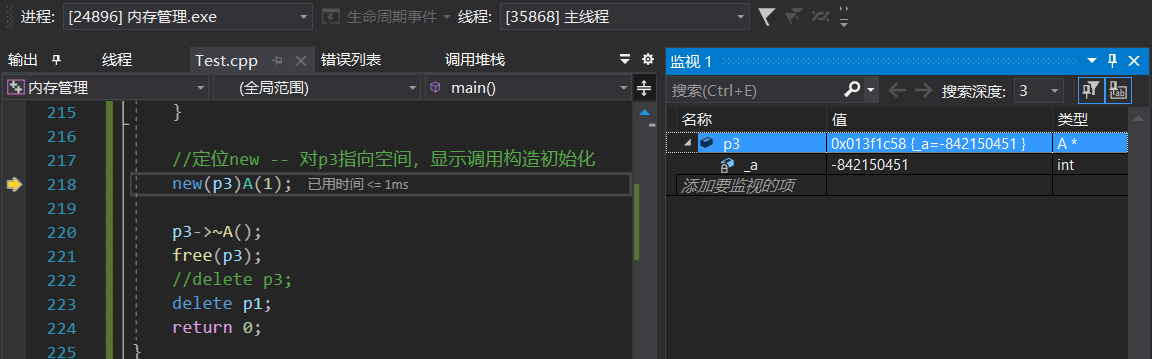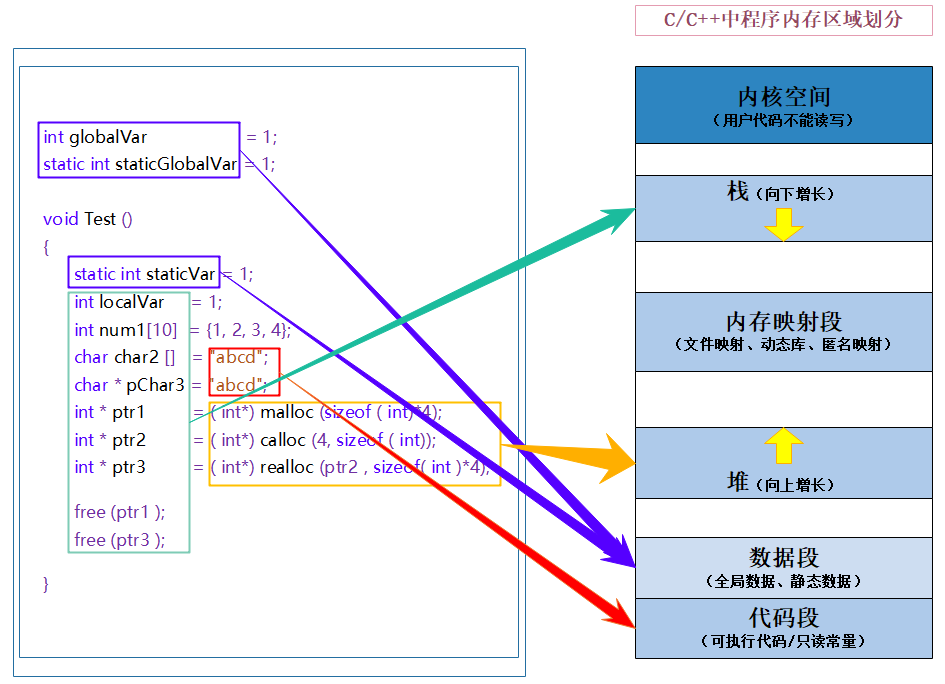# 经典分享 C++内存管理详解　２（共２部分）

+关注继续查看

5、杜绝“野指针”

“野指针”不是null指针，是指向“垃圾”内存的指针。人们一般不会错用null指针，因为用if语句很容易判断。但是“野指针”是很危险的，if语句对它不起作用。 “野指针”的成因主要有两种：

（1）指针变量没有被初始化。

1. char *p = null;
2. char *str = (char *) malloc(100);

（2）指针p被free或者delete之后，没有置为null，让人误以为p是个合法的指针。

（3）指针操作超越了变量的作用范围。

1. class a
2. {
3. 　public:
4. void func(void){ cout << “func of class a” << endl; }
5. };
6. void test(void)
7. {
8. 　a *p;
9. 　{
10. a a;
11. p = &a; // 注意 a 的生命期
12. 　}
13. 　p->func(); // p是“野指针”

6、有了malloc/free为什么还要new/delete？

malloc与free是c++/c语言的标准库函数，new/delete是c++的运算符。它们都可用于申请动态内存和释放内存。

1. class obj
2. {
3. 　public :
4. obj(void){ cout << “initialization” << endl; }
5. ~obj(void){ cout << “destroy” << endl; }
6. void initialize(void){ cout << “initialization” << endl; }
7. void destroy(void){ cout << “destroy” << endl; }
8. };
9. void usemallocfree(void)
10. {
11. 　obj *a = (obj *)malloc(sizeof(obj)); // 申请动态内存
12. 　a->initialize(); // 初始化
13. 　//…
14. 　a->destroy(); // 清除工作
15. 　free(a); // 释放内存
16. }
17. void usenewdelete(void)
18. {
19. 　obj *a = new obj; // 申请动态内存并且初始化
20. 　//…
21. 　delete a; // 清除并且释放内存
22. }
23. 　示例6 用malloc/free和new/delete如何实现对象的动态内存管理

7、内存耗尽怎么办？

（1）判断指针是否为null，如果是则马上用return语句终止本函数。例如：

1. void func(void)
2. {
3. 　a *a = new a;
4. 　if(a == null)
5. 　{
6. return;
7. 　}
8. 　…

（2）判断指针是否为null，如果是则马上用exit(1)终止整个程序的运行。例如：

1. void func(void)
2. {
3. 　a *a = new a;
4. 　if(a == null)
5. 　{
6. cout << “memory exhausted” << endl;
7. exit(1);
8. 　}
9. 　…

（3）为new和malloc设置异常处理函数。例如visual c++可以用_set_new_hander函数为new设置用户自己定义的异常处理函数，也可以让malloc享用与new相同的异常处理函数。

1. void main(void)
2. {
3. 　float *p = null;
4. 　while(true)
5. 　{
6. p = new float;
7. cout << “eat memory” << endl;
8. if(p==null)
9. 　exit(1);
10. 　}
11. }示例7试图耗尽操作系统的内存

8、malloc/free 的使用要点

1. void * malloc(size_t size);

1. int *p = (int *) malloc(sizeof(int) * length);

（1） malloc返回值的类型是void *，所以在调用malloc时要显式地进行类型转换，将void * 转换成所需要的指针类型。

（2） malloc函数本身并不识别要申请的内存是什么类型，它只关心内存的总字节数。我们通常记不住int, float等数据类型的变量的确切字节数。例如int变量在16位系统下是2个字节，在32位下是4个字节；而float变量在16位系统下是4个字节，在32位下也是4个字节。最好用以下程序作一次测试：

1. cout << sizeof(char) << endl;
2. cout << sizeof(int) << endl;
3. cout << sizeof(unsigned int) << endl;
4. cout << sizeof(long) << endl;
5. cout << sizeof(unsigned long) << endl;
6. cout << sizeof(float) << endl;
7. cout << sizeof(double) << endl;
8. cout << sizeof(void *) << endl;

（3）函数free的原型如下：

1. void free( void * memblock );

9、new/delete 的使用要点

1. int *p1 = (int *)malloc(sizeof(int) * length);
2. int *p2 = new int[length];

1. class obj
2. {
3. 　public :
4. obj(void); // 无参数的构造函数
5. obj(int x); // 带一个参数的构造函数
6. …
7. }
8. void test(void)
9. {
10. 　obj *a = new obj;
11. 　obj *b = new obj(1); // 初值为1
12. 　…
13. 　delete a;
14. 　delete b;

1. obj *objects = new obj; // 创建100个动态对象

1. obj *objects = new obj(1);// 创建100个动态对象的同时赋初值1

1. delete []objects; // 正确的用法
2. delete objects; // 错误的用法

10、一些心得体会

（1）越是怕指针，就越要使用指针。不会正确使用指针，肯定算不上是合格的程序员。

（2）必须养成“使用调试器逐步跟踪程序”的习惯，只有这样才能发现问题的本质。

【编辑推荐】C/C++内存管理

54 0C&C++内存管理
C&C++内存管理内容
59 0C/C++【内存管理】
C++中的内存管理机制和C语言是一样的，但在具体内存管理函数上，C语言的malloc已经无法满足C++面向对象销毁的需求，于是祖师爷在C++中新增了一系列内存管理函数，即 new 和 delete 著名段子：如果你还没没有对象，那就尝试 new 一个吧
43 0【C++修炼之路】6. 内存管理（二）
【C++修炼之路】6. 内存管理（二）
23 0【C++修炼之路】6. 内存管理（一）
【C++修炼之路】6. 内存管理（一）
26 0C++ 类和对象以及内存管理 练习错题总结
C++ 类和对象以及内存管理 练习错题总结
48 0

C++ 入门教程开发文档1714842